Courses

# RD Sharma Solutions - Ex-3.1, Decimals, Class 7, Math Class 7 Notes | EduRev

## Class 7 : RD Sharma Solutions - Ex-3.1, Decimals, Class 7, Math Class 7 Notes | EduRev

The document RD Sharma Solutions - Ex-3.1, Decimals, Class 7, Math Class 7 Notes | EduRev is a part of the Class 7 Course RD Sharma Solutions for Class 7 Mathematics.
All you need of Class 7 at this link: Class 7

Q1. Write each of the following as decimals:

Solution.

(i) 8/100

Mark the decimal point two places from right to left

= 8/100 = 0.08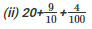Convert 9/10 and 4/100 into decimals

9/10

Mark the decimal point one place from right to left

9/10 = 0.9

4/100

Mark the decimal point two places from right to left

4/100

= 0.04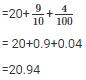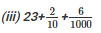Convert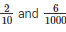into decimals

2/10

Mark the decimal point one place from right to left

2/10

= 0.2

6/1000

Mark the decimal point three places from right to left

6/1000

= 0.006

23, 0.2, 0.006 are unlike decimals. So we convert them into like decimals.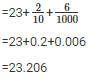Q2. Convert each of the following into fractions in the lowest form:

Solution.

(i) 0.04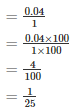(ii) 2.34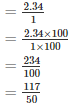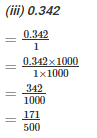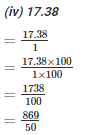Q3. Express the following fractions as decimals:

Solution.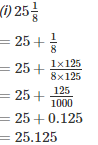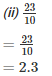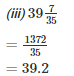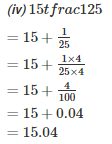Q4. What is to be added to 36.85 to get 59.41?

Solution.

x+36.85 = 59.41

x = 59.41-36.85

x = 22.56

Therefore 22.56 is added to 36.85 to get 59.41

Q5. What is to be subtracted from 17.1 to get 2.051?

Solution.

17.1-x = 2.051

17.1 = x+2.051

x = 17.1-2.051

x = 15.049

Therefore 15.049 is subtracted from 17.1 to get 15.049

Q6. By how much should 34.79 be increased to get 70.15?

Solution.

34.79+x = 70.15

x = 70.15-34.79

x = 35.36

Therefore 35.36 is increased to 70.15

Q7. By how much should 59.71 be decreased to get 34.58?

Solution.

59.71-x = 34.58

59.71-34.58 = x

x = 25.13

Therefore 25.13 is decreased to get 34.58

Offer running on EduRev: Apply code STAYHOME200 to get INR 200 off on our premium plan EduRev Infinity!

97 docs

,

,

,

,

,

,

,

,

,

,

,

,

,

,

,

,

,

,

,

,

,

,

,

,

,

,

,

,

,

,

;